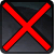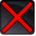# [grant_valor_rankup_reward]Conditions

## Related effects, buffs and debuffs

• [hidden] [1s]Effect #1

 Slot: Buff Duration: 1s Tick rate: does not tick # occurrences: 0
• On Apply

Perform the following actions:

• Play appearance epp . pvp . grant_valor_rank, dependent on calling effect
• On Apply

Only when the following conditions are met:

• If valor rank is = 10

Perform the following actions:

• Execute Hydra Hook
- Event Name = (string) rank_10
- Object Self = (int) 1
• On Apply

Only when the following conditions are met:

• If valor rank is = 20

Perform the following actions:

• Execute Hydra Hook
- Event Name = (string) rank_20
- Object Self = (int) 1
• On Apply

Only when the following conditions are met:

• If valor rank is = 20

Perform the following actions:

• Execute Hydra Hook
- Event Name = (string) rank_20
- Object Self = (int) 1
• On Apply

Only when the following conditions are met:

• If valor rank is = 30

Perform the following actions:

• Execute Hydra Hook
- Event Name = (string) rank_30
- Object Self = (int) 1
• On Apply

Only when the following conditions are met:

• If valor rank is = 40

Perform the following actions:

• Execute Hydra Hook
- Event Name = (string) rank_40
- Object Self = (int) 1
• On Apply

Only when the following conditions are met:

• If valor rank is = 50

Perform the following actions:

• Execute Hydra Hook
- Event Name = (string) rank_50
- Object Self = (int) 1
• On Apply

Only when the following conditions are met:

• If valor rank is = 60

Perform the following actions:

• Execute Hydra Hook
- Event Name = (string) rank_60
- Object Self = (int) 1
• On Apply

Only when the following conditions are met:

• If valor rank is = 70

Perform the following actions:

• Execute Hydra Hook
- Event Name = (string) rank_70
- Object Self = (int) 1
• On Apply

Only when the following conditions are met:

• If valor rank is = 80

Perform the following actions:

• Execute Hydra Hook
- Event Name = (string) rank_80
- Object Self = (int) 1
• On Apply

Only when the following conditions are met:

• If valor rank is = 90

Perform the following actions:

• Execute Hydra Hook
- Event Name = (string) rank_90
- Object Self = (int) 1
• On Apply

Only when the following conditions are met:

• If valor rank is = 100

Perform the following actions:

• Execute Hydra Hook
- Event Name = (string) rank_100
- Object Self = (int) 1